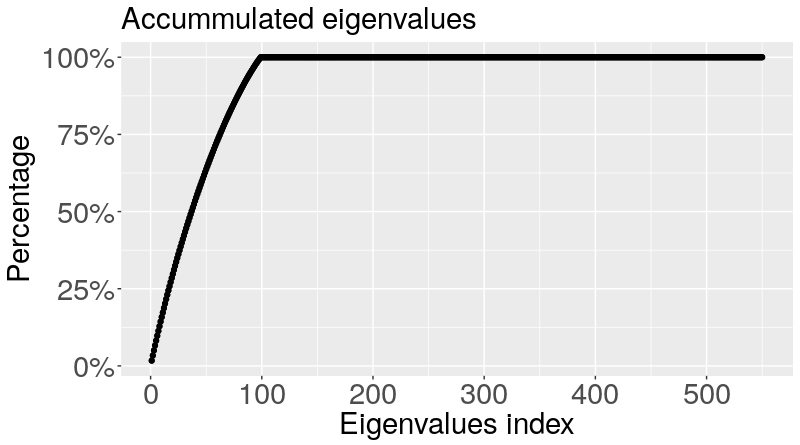# Motivation

I remember one lecture about linear models in the University of Costa Rica. He said before to present some classic method “This only works if you have more data than variables”. In this moment, it seems very reasonable and I could not imagine any real case with more variables ($p$) than data ($n$).

I was wrong!.

Nowadays we have more and more datasets with $p\gg n$. For example in climate problems, finance, voice recognition, among others. All these examples share that the number of observations is less than the number of characteristics that we want to study.

Let $X\in\R^p$ and $\Sigma=\Cov(X)$. The first idea that comes in mind with such problems it to reduce the dimensionality. Thus, the classic procedure is starting by the study of the eigenvectors of $\Sigma$ and respectively its eigenspace. The study of the eigenspace serves as basis for other techniques such as principal component analysis.

# Data, variables and eigenspaces

One interesting question is: How much impact the difference between $n$ and $p$ the estimation of $\Sigma$?

Short answer: A lot! We can have issues on lack of consistency, spreading of the eigenvalues, among others.

Assume that we observe $X_1,\ldots, X_n$ and i.i.d. sample of $X$ with covariance matrix $\Sigma$. The sample covariance matrix for $X_1,\ldots, X_n$ is

\begin{equation*}
\hat{\Sigma} = \frac{1}{n}\sum_{i=1}^n (X_i – \bar{X})(X_i –
\bar{X})^\top.
\end{equation*}

By classic theory in asymptotic statistics, if $p$ is fixed, $\hat{\Sigma}$ converges at rate $n^{-1/2}$. Otherwise, we will lost the consistency of the estimator by having non-convergent rates. For example under the Frobenius norm we have that

\begin{equation*}
\Vert \hat{\Sigma} -\Sigma \Vert_F \leq \frac{p} {n}.
\end{equation*}

So notice that when $p$ goes to infinity faster than $n$, we cannot longer ensure the convegence of $\hat{\Sigma}$.

Moreover, assume that $X\sim N(0,\Sigma=I_p)$ and $p/n\to c\in (0,1)$. Thus, the distribution of $\hat{\Sigma}$ follows a Marĉenko-Pasteur law supported on $((1-\sqrt{c})^2, (1+\sqrt{c})^2)$. It means, the larger $p/n$ the more spread out of the eigenvalues. For a detailed discussion about the subject refer to  and .

To illustrate the last point I took 100 multivariate normal random variables with mean 0 and identity covariance. Next, I estimate the empirical covariance and its eigenvalues. Finally, I created the proportion of accumulated eigenvalues with respect to its sum.

The following plot show us that we only need 100 variables to explain the 99.99% of the variance. In other words, we have 900 variables of pure noise in the model.Accumulated eigenvalues taken from a 100 samples of a $\mathcal{N}_{1000}(0,\mathrm{I})$

Summarizing: If $p\to \infty$ we need to regularize $\hat{\Sigma}$ to avoid strange behaviors in our analyses.

What can we do to solve those issues?

I will present you three techniques to regularize the matrix $\hat{\Sigma}$ that I recently have studied. Please, let me know in the comments if you know or are working on others.

In all the cases I will use $\Vert \cdot \Vert$ to denote the operator norm and $\Vert \cdot \Vert_F$ the Frobenius norm.

# Banding technique

In , the authors present a banding estimator which for any $0\leq k \leq p$,

\begin{equation*}
\hat{\Sigma}_{k,banding} = [\hat{\sigma}_{ij}\ \mathbf{1}(\vert
i-j\vert\leq k)]
\end{equation*}

The main result in this paper is the following,

Theorem 1. If $X$ is Gaussian and $\mathcal{U}(\varepsilon_0,\alpha,C)$ is a class of regular covariance matrices. Then if $k \asymp (n^{-1}\log{p})^{-1/(2(\alpha+1))}$

\begin{equation*}
\Vert \hat{\Sigma}_{k,banding} -\Sigma \Vert =O_p \left( \left(
\frac{\log p}{n} \right)^{\alpha/(2(\alpha+1))} \right)
\end{equation*}

uniformly on $\Sigma \in \mathcal{U}$

It is not possible assure the $\hat{\Sigma}_{k,banding}$ positive definiteness. Instead, they propose a tapering estimator via the Schur (coordinate-wise) matrix multiplication.

# Thresholding techniques

In the same year, the article  gives a hard thresholding estimator for $\Sigma$. For any $0\leq s \leq \infty$, define

\begin{equation*}
\hat{\Sigma}_{k,threshold} = [\hat{\sigma}_{ij} \ \mathbf{1}(\vert
\hat{\sigma}_{ij} \vert\geq s)].
\end{equation*}

$\hat{\Sigma}_{k,threshold}$ preserve symmetry but is not necessary positive definite. However if

\begin{equation*}
\Vert \hat{\Sigma}_{k,thresold} – \hat{\Sigma}_{0,threshold} \Vert
\varepsilon
\end{equation*}

then $\hat{\Sigma}_{k,threshold}$ is necessarily positive definite, since for all vectors $v$ with $\Vert v \Vert_2 = 1$ we have $v^\top\hat{\Sigma}_kv\geq v^\top\Sigma v \leq \lambda_{min} -\varepsilon$.

Theorem 2. Suppose $X$ gaussian. Uniformly on some class of covariance matrices, $\mathcal{U}_\tau(q, c_0(p),M)$, for sufficient large $M^\prime$, if

\begin{equation*}
s_n = M^\prime \sqrt{\frac{\log p}{n}}
\end{equation*}

and $\log p /n =o(1)$, then

\begin{equation*}
\Vert\hat{\Sigma}_{s_n,threshold} -\Sigma\Vert^2 = O_p \left( c_0(p)
\left( \frac{\log p}{n} \right)^{(1-q)/2} \right).
\end{equation*}

Moreover,

\begin{equation*}
\Vert\hat{\Sigma}_{s_n,threshold} -\Sigma\Vert_F^2 = O_p \left( c_0(p)
\left( \frac{\log p}{n} \right)^{1-q/2} \right).
\end{equation*}

## Comparative with the banding estimator

They claim that the banding estimator is slightly better if $q>\frac{1}{\alpha+1}$. Thus,

\begin{equation*}
O_p \left( \left( \frac{\log p}{n} \right)^{\alpha/(2(\alpha+1)) }
\right)\leq O_p \left( \left( \frac{\log p}{n} \right)^{(1-q)/2}
\right).
\end{equation*}

In the genuine sparse case ($\alpha\to \infty$), both rates approach to $\left(\frac{\log p}{n}\right)^{1/2}$.

# Tapering technique

Finally, in  they implemented the tapering estimator suggested by "Peter J. Bickel and Elizaveta Levina" (). Formally,

\begin{equation*}
\hat{\Sigma}_{k,tapering} = (w_{ij}\hat{\sigma}_{ij})_{p\times p}
\end{equation*}

where $k_h = k/2$

\begin{equation*}
w_{ij}=
\begin{cases}
1,& \text{when } \vert i-j \vert \leq k_h\\
2-\frac{\vert i-j \vert}{k_h}, &
\text{when } k_h \leq \vert i-j \vert \leq k\\
0,& \text{otherwise }
\end{cases}
\end{equation*}

Again the estimator could have negative eigenvalues. They propose first diagonalize $\hat{\Sigma}$ and then replace them by 0.

They also prove an optimal rate from the minimax point of view.

Theorem 3. The minimax risk of estimating the covariance matrix $\Sigma$ over a covariance matrix class $\mathcal{P}_\alpha$ satisfies,

\begin{equation*}
\inf_{\Sigma_k}\sup_{\mathcal{P}_\alpha} \mathbb{E} \Vert
\hat{\Sigma}_{k,tapering} -\Sigma \Vert^2 \asymp \min\left\{
n^{-2\alpha / (2\alpha+1)} + \frac{\log p }{n},
\frac{p}{n}\right\}.
\end{equation*}

The minimax risk over the Frobenius norm and $\mathcal{P}^\prime_\alpha$ another covariance matrices class, satisfies,

\begin{equation*}
\inf_{\Sigma_k}\sup_{\mathcal{P}^\prime_\alpha} \mathbb{E} \Vert
\hat{\Sigma}_{k,tapering} -\Sigma \Vert_F^2 \asymp \min\left\{
n^{-(2\alpha+1) / (2(\alpha+1))}, \frac{p}{n}\right\}.
\end{equation*}

The main difficulty on these method is the lack of positive definiteness of $\hat{\Sigma}$. Theoretically, $\hat{\Sigma}$ converges in probability to a positive definite matrix $\Sigma$. However, in the practice, we will find small negative eigenvalue in our estimations. As a rule of thumb, we can simply replace the negative eigenvalues by zero without lose a lot of information, but of course, it will depend in each case.